Get instant live expert help with Excel or Google Sheets“My Excelchat expert helped me in less than 20 minutes, saving me what would have been 5 hours of work!”

#### Post your problem and you’ll get expert help in seconds.

Your message must be at least 40 characters
Our professional experts are available now. Your privacy is guaranteed.

# Pad week numbers with zerosRead time: 20 minutes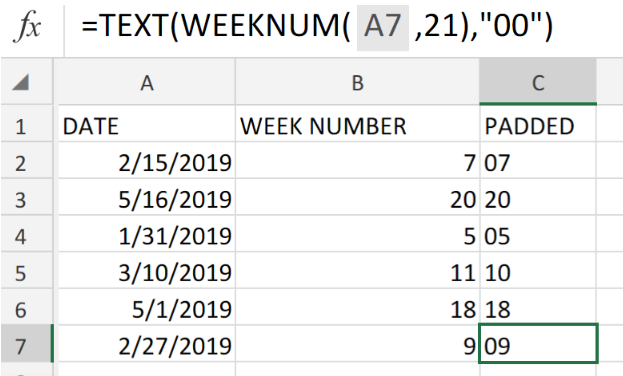Figure 1. Pad Week Numbers With Zeros in Excel.

If we want to pad week numbers with zeros in Excel, we have to make use of the TEXT function.

## Generic Formula

`=TEXT(WEEKNUM(date,type),"00")`

With the Excel TEXT function, we can “pad” any number value with as many zeros as we like.

## How to use the TEXT Function in Excel.

We are now going to demonstrate the use of the TEXT Function using 3 simple steps.

1. Collect the calendar dates to be padded and their corresponding week numbers and arrange within properly labeled columns of our worksheet.

Be sure to provide another labeled column with empty cells for Excel to return the padded number values.

See example illustrated below;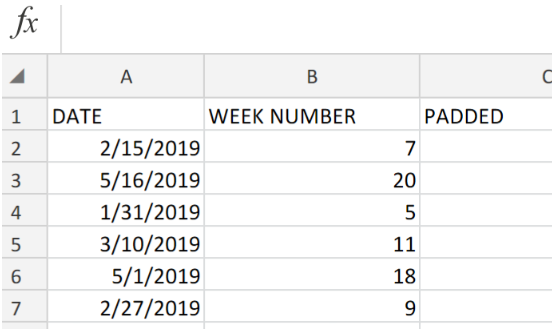Figure 2. Pad Week Numbers With Zeros in Excel.

1. In our worksheet example above, the TEXT Function we are going to enter into cell C2 is as follows;

`=TEXT(WEEKNUM(A2,21),"00")`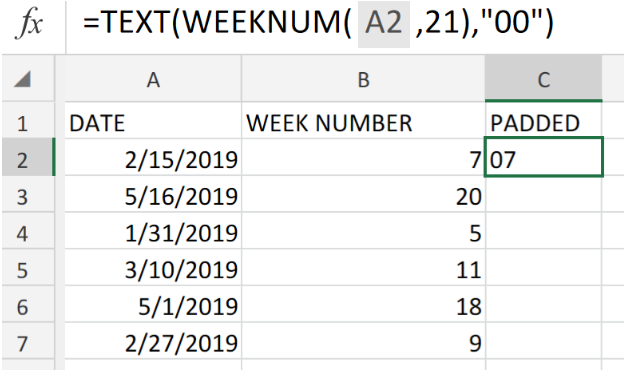Figure 3. Pad Week Numbers With Zeros in Excel.

Excel returned “07” as the padded result for the date in cell A2.

1. Copy the modified version of the TEXT Formula in cell C2 down into the other cells in the PADDED column.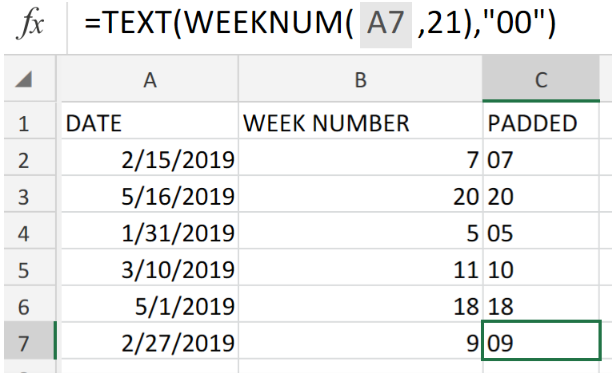Figure 4. Pad Week Numbers With Zeros in Excel.

The TEXT Function in Excel allows us to apply any kind of number formats, including percentage, currency, date, etc.

We can “pad” numbers with any amount of zeros we would like by applying number formats such as, “00”, “000”, “0000”.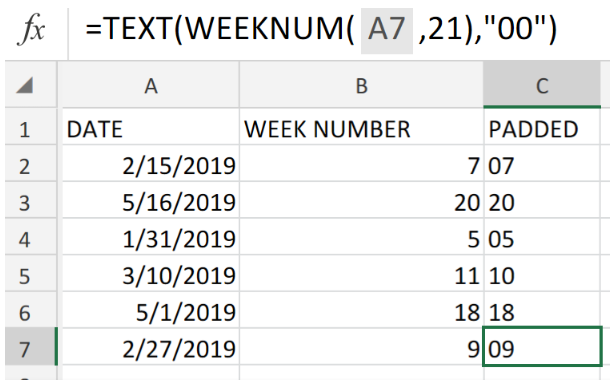Figure 5. Final Result.

## Instant Connection to an Expert through our Excelchat Service:

Our live Excelchat Service is here for you. We have Excel Experts available 24/7 to answer any Excel questions you may have. Guaranteed connection within 30 seconds and a customized solution for you within 20 minutes.

### Did this post not answer your question? Get a solution from connecting with the expert.Another blog reader asked this question today on Excelchat:
Related blogs
Solution examplesGood morning, I need a cell formatting solution to display leading zero in my excel table with the following conditions. 1. Cannot format as text 2. cell size is not fixed. Please let me know if there is a solution.Thanks in advance
Solved by V. C. in 11 minslooking for an Iferror formula that will bring back a blank value if a date is not listed. Currently using the following but want to add an Iferror statement: =TEXT(H2,"DDDD"). H2 is the raw date ex. 2/8/18. I tried =IFERROR(TEXT(H2,"DDDD"),"") but it keeps returning "saturday" instead of a blank cell. Any help is appreciated.
Solved by F. Q. in 20 minsHow do I make this formula =Value(IFerror(TEXT(AM3,"HMM"),"0")) Return a value of 2400 if the value is not an error?
Solved by F. J. in 21 minsI have an excel spreadsheet with two worksheets. And the following formula is not pulling the data. =VLOOKUP(\$A\$2:\$A\$566,'Module Type Info'!\$A\$2:\$D\$97,4,FALSE) In sheet 1, I am using all data in column 1 for the lookup In sheet 2, I have selected the first 4 columns of data for array I want to pull data from sheet 2, column 4 into sheet 1 Both tabs are sorted alphabetically. Confirmed that the value in column 4 of sheet 2 is a TEXT field. Why is this not pulling the info from sheet 2 into sheet 1
Solved by F. L. in 39 minsI need to add an formula into this one: =IF(\$L\$1="","",VLOOKUP(TEXT(\$L\$1,"000000"),'FRS102 BASE & GBP TB MAY 2018'!\$E:\$X,MATCH(A25,'FRS102 BASE & GBP TB MAY 2018'!\$Q\$3:\$X\$3,0)+3,0))
Solved by K. D. in 11 mins## Subscribe to Excelchat.coAnother blog reader asked this question today on Excelchat: